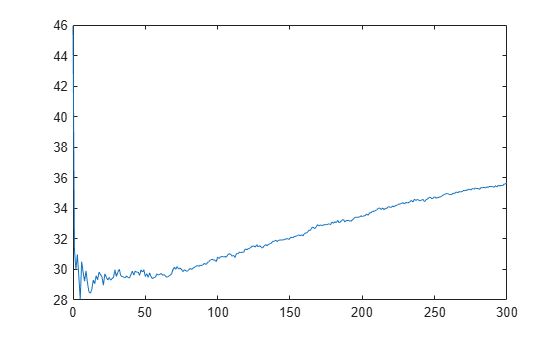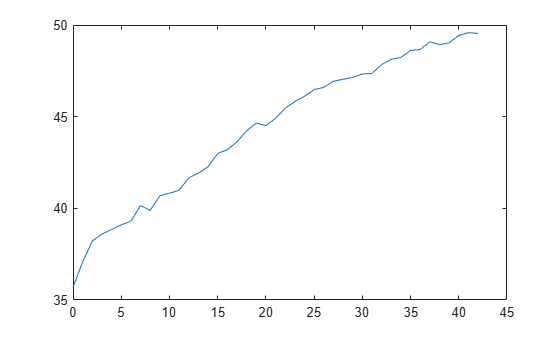Main Content

# RegressionPartitionedGAM

Cross-validated generalized additive model (GAM) for regression

## Description

`RegressionPartitionedGAM` is a set of generalized additive models trained on cross-validated folds. Estimate the quality of the cross-validated regression by using one or more kfold functions: `kfoldPredict`, `kfoldLoss`, and `kfoldfun`.

Every kfold object function uses models trained on training-fold (in-fold) observations to predict the response for validation-fold (out-of-fold) observations. For example, suppose you cross-validate using five folds. The software randomly assigns each observation into five groups of equal size (roughly). The training fold contains four of the groups (roughly 4/5 of the data), and the validation fold contains the other group (roughly 1/5 of the data). In this case, cross-validation proceeds as follows:

1. The software trains the first model (stored in `CVMdl.Trained{1}`) by using the observations in the last four groups, and reserves the observations in the first group for validation.

2. The software trains the second model (stored in `CVMdl.Trained{2}`) by using the observations in the first group and the last three groups. The software reserves the observations in the second group for validation.

3. The software proceeds in a similar manner for the third, fourth, and fifth models.

If you validate by using `kfoldPredict`, the software computes predictions for the observations in group i by using the ith model. In short, the software estimates a response for every observation by using the model trained without that observation.

## Creation

You can create a `RegressionPartitionedGAM` model in two ways:

• Create a cross-validated model from a GAM object `RegressionGAM` by using the `crossval` object function.

• Create a cross-validated model by using the `fitrgam` function and specifying one of the name-value arguments `'CrossVal'`, `'CVPartition'`, `'Holdout'`, `'KFold'`, or `'Leaveout'`.

## Properties

expand all

### Cross-Validation Properties

This property is read-only.

Cross-validated model name, specified as `'GAM'`.

This property is read-only.

Number of cross-validated folds, specified as a positive integer.

Data Types: `double`

This property is read-only.

Cross-validation parameter values, specified as an object. The parameter values correspond to the values of the name-value arguments used to cross-validate the generalized additive model. `ModelParameters` does not contain estimated parameters.

You can access the properties of `ModelParameters` using dot notation.

This property is read-only.

Data partition indicating how the software splits the data into cross-validation folds, specified as a `cvpartition` model.

This property is read-only.

Compact models trained on cross-validation folds, specified as a cell array of `CompactRegressionGAM` model objects. `Trained` has k cells, where k is the number of folds.

Data Types: `cell`

### Other Regression Properties

This property is read-only.

Categorical predictor indices, specified as a vector of positive integers. `CategoricalPredictors` contains index values corresponding to the columns of the predictor data that contain categorical predictors. If none of the predictors are categorical, then this property is empty (`[]`).

Data Types: `double`

This property is read-only.

Number of observations in the training data stored in `X` and `Y`, specified as a numeric scalar.

Data Types: `double`

This property is read-only.

Predictor variable names, specified as a cell array of character vectors. The order of the elements of `PredictorNames` corresponds to the order in which the predictor names appear in the training data.

Data Types: `cell`

This property is read-only.

Response variable name, specified as a character vector.

Data Types: `char`

Response transformation function, specified as `'none'` or a function handle. `ResponseTransform` describes how the software transforms raw response values.

For a MATLAB® function or a function that you define, enter its function handle. For example, you can enter ```Mdl.ResponseTransform = @function```, where `function` accepts a numeric vector of the original responses and returns a numeric vector of the same size containing the transformed responses.

Data Types: `char` | `function_handle`

This property is read-only.

Observation weights used to train the model, specified as an n-by-1 numeric vector. n is the number of observations (`NumObservations`).

The software normalizes the observation weights specified in the `'Weights'` name-value argument so that the elements of `W` sum up to 1.

Data Types: `double`

This property is read-only.

Predictors used to cross-validate the model, specified as a numeric matrix or table.

Each row of `X` corresponds to one observation, and each column corresponds to one variable.

Data Types: `single` | `double` | `table`

This property is read-only.

Response used to cross-validate the model, specified as a numeric vector.

Each row of `Y` represents the observed response of the corresponding row of `X`.

Data Types: `single` | `double`

## Object Functions

 `kfoldPredict` Predict responses for observations in cross-validated regression model `kfoldLoss` Loss for cross-validated partitioned regression model `kfoldfun` Cross-validate function for regression

## Examples

collapse all

Train a cross-validated GAM with 10 folds, which is the default cross-validation option, by using `fitrgam`. Then, use `kfoldPredict` to predict responses for validation-fold observations using a model trained on training-fold observations.

Load the `carbig` data set, which contains measurements of cars made in the 1970s and early 1980s.

`load carbig`

Create a table that contains the predictor variables (`Acceleration`, `Displacement`, `Horsepower`, and `Weight`) and the response variable (`MPG`).

`tbl = table(Acceleration,Displacement,Horsepower,Weight,MPG);`

Create a cross-validated GAM by using the default cross-validation option. Specify the `'CrossVal'` name-value argument as `'on'`.

```rng('default') % For reproducibility CVMdl = fitrgam(tbl,'MPG','CrossVal','on')```
```CVMdl = RegressionPartitionedGAM CrossValidatedModel: 'GAM' PredictorNames: {1x4 cell} ResponseName: 'MPG' NumObservations: 398 KFold: 10 Partition: [1x1 cvpartition] NumTrainedPerFold: [1x1 struct] ResponseTransform: 'none' Properties, Methods ```

The `fitrgam` function creates a `RegressionPartitionedGAM` model object `CVMdl` with 10 folds. During cross-validation, the software completes these steps:

1. Randomly partition the data into 10 sets.

2. For each set, reserve the set as validation data, and train the model using the other 9 sets.

3. Store the 10 compact, trained models a in a 10-by-1 cell vector in the `Trained` property of the cross-validated model object `RegressionPartitionedGAM`.

You can override the default cross-validation setting by using the `'CVPartition'`, `'Holdout'`, `'KFold'`, or `'Leaveout' `name-value argument.

Predict responses for the observations in `tbl` by using `kfoldPredict`. The function predicts responses for every observation using the model trained without that observation.

`yHat = kfoldPredict(CVMdl);`

`yHat` is a numeric vector. Display the first five predicted responses.

`yHat(1:5)`
```ans = 5×1 19.4848 15.7203 15.5742 15.3185 17.8223 ```

Compute the regression loss (mean squared error).

`L = kfoldLoss(CVMdl)`
```L = 17.7248 ```

`kfoldLoss` returns the average mean squared error over 10 folds.

Train a regression generalized additive model (GAM) by using `fitrgam`, and create a cross-validated GAM by using `crossval` and the holdout option. Then, use `kfoldPredict` to predict responses for validation-fold observations using a model trained on training-fold observations.

Load the `patients` data set.

`load patients`

Create a table that contains the predictor variables (`Age`, `Diastolic`, `Smoker`, `Weight`, `Gender`, `SelfAssessedHealthStatus`) and the response variable (`Systolic`).

`tbl = table(Age,Diastolic,Smoker,Weight,Gender,SelfAssessedHealthStatus,Systolic);`

Train a GAM that contains linear terms for predictors.

`Mdl = fitrgam(tbl,'Systolic');`

`Mdl` is a `RegressionGAM` model object.

Cross-validate the model by specifying a 30% holdout sample.

```rng('default') % For reproducibility CVMdl = crossval(Mdl,'Holdout',0.3)```
```CVMdl = RegressionPartitionedGAM CrossValidatedModel: 'GAM' PredictorNames: {1x6 cell} CategoricalPredictors: [3 5 6] ResponseName: 'Systolic' NumObservations: 100 KFold: 1 Partition: [1x1 cvpartition] NumTrainedPerFold: [1x1 struct] ResponseTransform: 'none' Properties, Methods ```

The `crossval` function creates a `RegressionPartitionedGAM` model object `CVMdl` with the holdout option. During cross-validation, the software completes these steps:

1. Randomly select and reserve 30% of the data as validation data, and train the model using the rest of the data.

2. Store the compact, trained model in the `Trained` property of the cross-validated model object `RegressionPartitionedGAM`.

You can choose a different cross-validation setting by using the `'CrossVal'`, `'CVPartition'`, `'KFold'`, or `'Leaveout' `name-value argument.

Predict responses for the validation-fold observations by using `kfoldPredict`. The function predicts responses for the validation-fold observations by using the model trained on the training-fold observations. The function assigns `NaN` to the training-fold observations.

`yFit = kfoldPredict(CVMdl);`

Find the validation-fold observation indexes, and create a table containing the observation index, observed response values, and predicted response values. Display the first eight rows of the table.

```idx = find(~isnan(yFit)); t = table(idx,tbl.Systolic(idx),yFit(idx), ... 'VariableNames',{'Obseraction Index','Observed Value','Predicted Value'}); head(t)```
```ans=8×3 table Obseraction Index Observed Value Predicted Value _________________ ______________ _______________ 1 124 130.22 6 121 124.38 7 130 125.26 12 115 117.05 20 125 121.82 22 123 116.99 23 114 107 24 128 122.52 ```

Compute the regression error (mean squared error) for the validation-fold observations.

`L = kfoldLoss(CVMdl)`
```L = 43.8715 ```

Train a cross-validated generalized additive model (GAM) with 10 folds. Then, use `kfoldLoss` to compute the cumulative cross-validation regression loss (mean squared errors). Use the errors to determine the optimal number of trees per predictor (linear term for predictor) and the optimal number of trees per interaction term.

Alternatively, you can find optimal values of `fitrgam` name-value arguments by using the `bayesopt` function. For an example, see Optimize Cross-Validated GAM Using bayesopt.

Load the `patients` data set.

`load patients`

Create a table that contains the predictor variables (`Age`, `Diastolic`, `Smoker`, `Weight`, `Gender`, and `SelfAssessedHealthStatus`) and the response variable (`Systolic`).

`tbl = table(Age,Diastolic,Smoker,Weight,Gender,SelfAssessedHealthStatus,Systolic);`

Create a cross-validated GAM by using the default cross-validation option. Specify the `'CrossVal'` name-value argument as `'on'`. Also, specify to include 5 interaction terms.

```rng('default') % For reproducibility CVMdl = fitrgam(tbl,'Systolic','CrossVal','on','Interactions',5);```

If you specify `'Mode'` as `'cumulative'` for `kfoldLoss`, then the function returns cumulative errors, which are the average errors across all folds obtained using the same number of trees for each fold. Display the number of trees for each fold.

`CVMdl.NumTrainedPerFold `
```ans = struct with fields: PredictorTrees: [300 300 300 300 300 300 300 300 300 300] InteractionTrees: [76 100 100 100 100 42 100 100 59 100] ```

`kfoldLoss` can compute cumulative errors using up to 300 predictor trees and 42 interaction trees.

Plot the cumulative, 10-fold cross-validated, mean squared errors. Specify `'IncludeInteractions'` as `false` to exclude interaction terms from the computation.

```L_noInteractions = kfoldLoss(CVMdl,'Mode','cumulative','IncludeInteractions',false); figure plot(0:min(CVMdl.NumTrainedPerFold.PredictorTrees),L_noInteractions)```The first element of `L_noInteractions` is the average error over all folds obtained using only the intercept (constant) term. The (`J+1`)th element of `L_noInteractions` is the average error obtained using the intercept term and the first `J` predictor trees per linear term. Plotting the cumulative loss allows you to monitor how the error changes as the number of predictor trees in the GAM increases.

Find the minimum error and the number of predictor trees used to achieve the minimum error.

`[M,I] = min(L_noInteractions)`
```M = 28.0506 ```
```I = 6 ```

The GAM achieves the minimum error when it includes 5 predictor trees.

Compute the cumulative mean squared error using both linear terms and interaction terms.

```L = kfoldLoss(CVMdl,'Mode','cumulative'); figure plot(0:min(CVMdl.NumTrainedPerFold.InteractionTrees),L)```The first element of `L` is the average error over all folds obtained using the intercept (constant) term and all predictor trees per linear term. The (`J+1`)th element of `L` is the average error obtained using the intercept term, all predictor trees per linear term, and the first `J` interaction trees per interaction term. The plot shows that the error increases when interaction terms are added.

If you are satisfied with the error when the number of predictor trees is 5, you can create a predictive model by training the univariate GAM again and specifying `'NumTreesPerPredictor',5` without cross-validation.

## See Also

Introduced in R2021a

## Support

#### Mastering Machine Learning: A Step-by-Step Guide with MATLAB

Download ebook